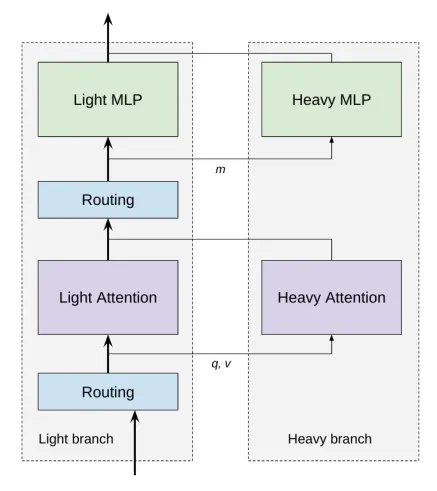• 为何上下文长度如此重要，且能在LLM中起到举足轻重的作用？
• 处理大型上下文长度时，原始Transformer架构的主要局限性是什么？
• Transformer架构的计算复杂度
• 目前有哪些可以加速Transformer并将上下文长度增加到100K的优化技术？

## 重点概览

• 第一个问题是注意力层（attention layer）计算的二次方时间（Quadratic time）和空间复杂度，即输入tokens数量$n$

• 当embedding大小$d>n$时，第二个问题是embedding大小$d$的线性层的二次方时间复杂度。

• 第三个问题是原始架构中使用的位置正弦嵌入（Positional Sinusoidal Embedding ）。

• 在Transformer架构中，可学习（learnable）矩阵权重的形状与输入tokens个数$n$无关。

• 因此，在2K上下文长度中训练的Transformer可以使用任意长度的tokens，甚至是100K个tokens。但如果不是在100K个tokens上训练出来的，那么该模型在100K个tokens的推理过程中不会产生有意义的推理结果。

• 由于$n$$d$相关的二次复杂度，在大型语料库上训练Vanilla Transformer，并且只在较大的上下文长度上训练是不可行的。据估计，在2K上下文长度上训练LLaMA的费用约为300万美元，因此，100K的花费约为1.5亿美元。

• 一种选择是，可以在2K个tokens上下文中训练模型，然后在更长的上下文tokens（例如65K）中微调。但由于位置正弦编码（Positional Sinusoidal Encoding）的存在，这不适用于原始Transformer模型。

• [技巧1] 为解决此问题，可删除位置正弦编码并使用ALiBi，参考苏神博客，这一简单位置嵌入不会影响准确性。然后可以在2K个tokens上训练，在100K个tokens上微调。

• [技巧2] 无需计算所有tokens间的注意力分数（attention scores）。某些tokens比其他tokens更重要，因此可使用稀疏注意力。这将提升训练和推理速度。

• [技巧3] Flash Attention有效地实现了GPU的注意力层。它使用切片（tiling）技术，避免生成不适合GPU SRAM容量的大型中间矩阵$（n，n）$。这将提升训练和推理速度。

• [技巧4] 选择多查询注意力（Multi-Query attention），而非多头注意力。这意味着线性投影$K$$V$时，可在跨所有注意力头（head）中共享权重。这极大地加快了增量（incremental）推理速度。

• [技巧5] 条件计算（Conditional computation）避免将所有模型参数应用于输入序列中的所有tokens。CoLT5仅对最重要的tokens应用重量级计算，并使用较轻量级的层处理其余tokens。这将加速训练和推理。

• [技巧6] 为适应大型上下文，需要GPU中有大量RAM，因此人们使用80GB的A100 GPU。

## 为何上下文长度如此重要？

• 尝试总结技巧和复杂的链式提示。

• 维护向量数据库以保留自定义文档的嵌入，然后通过相似性指标在它们之间展开“搜索”。

• 尽可能使用自定义数据微调LLM（并非所有商业LLM都允许自定义微调，对开源LLM进行自定义微调并不常见）。

• 为特定数据开发定制小型LLM（同样，这并非常规任务）

## 多头注意力回顾

$Q$ - 查询（query），$K$ - 键（key），$V$ - 值（value），这些符号是论文中与信息检索相关的符号表示法。在信息检索中，你可以将一个“查询”输入系统，并搜索与之最接近的“键”。

$n$ - 输入的tokens数量

$d$ - 文本嵌入维度

$h$ - 注意力头的数量

$k$ -$Q$$K$的线性投影大小

$v$ -$V$的线性投影大小

1. 我们有一个Embedding层，用于接收tokens作为输入，并返回大小为$（1，d）$的向量。因此，对于一个由$n$个tokens组成的序列，我们得到大小为$（n，d）$的文本嵌入矩阵$X$，然后将其与位置正弦嵌入相加。

2. 多头注意力层旨在为tokens序列计算新的嵌入表示，该tokens序列可以被视为对原始文本编码$X$，但需要，（1）根据tokens间相对于上下文的重要性进行加权，（2）根据tokens的相对位置进行加权。

3. 我们使用$h$个注意力头对嵌入矩阵$X（n×d）$进行并行处理。为了使所有的注意力头都得到$Q$$K$$V$，我们需要对$X$进行线性投影，将其分别投影到$k$$k$$v$维度。为此，可以通过将$X$分别与形状为$（d，k）$$（d，k）$$（d，v）$$h$个矩阵相乘来实现。你可将其理解为用$（n，d）$乘以$（h，d，k）$$（h，d，k）$$（h，d，v）$

4. 注意力头返回大小为$（n，v）$$h$个注意力分数矩阵。然后，我们将来自所有注意力头$（n，h*v）$的片段进行连接，并对其进行线性投影，为后续步骤做准备。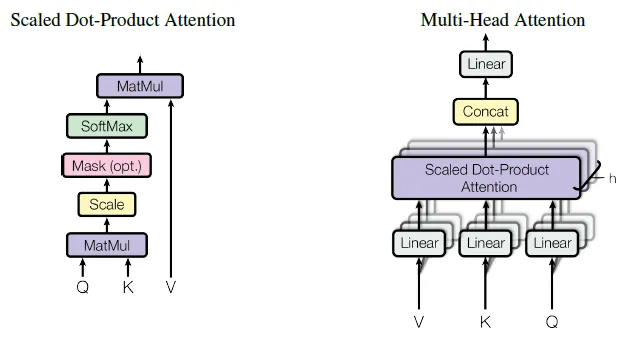### 缩放点积注意力（Scaled Dot-Product Attention）

1. $Q$$K$$V$$X$的3个线性投影，大小分别为$（n，k）$$（n，k）$$（n，v）$，通过乘以每个注意力头的可学习权重（learnable weight）获得。

2. 通过计算$Q$$K$（转置）之间的距离（点积），我们得到了注意力分数。将矩阵$（n，k）$$（k，n）$相乘，得到矩阵$（n，n）$，然后我们将其与掩码矩阵相乘，以将一些tokens置零（在解码器中需要）。接下来，我们对其进行缩放，并应用softmax函数，使注意力分数范围在0到1之间。这样，我们就得到一个形状为$（n，n）$的矩阵，其中$n_ij$表示第$i$个和第$j$个token之间的相对注意力分数（0-1之间），这展示了这些tokens在给定长度为n的特定上下文中有多“接近（close）”。

3. 然后，我们将这个注意力分数矩阵$（n，n）$乘以大小为$（n，d）$的“值（value）”$V$，以获得由这些相对注意力分数加权得到的文本嵌入。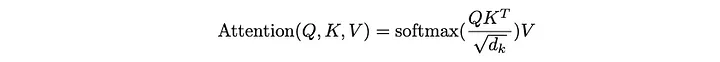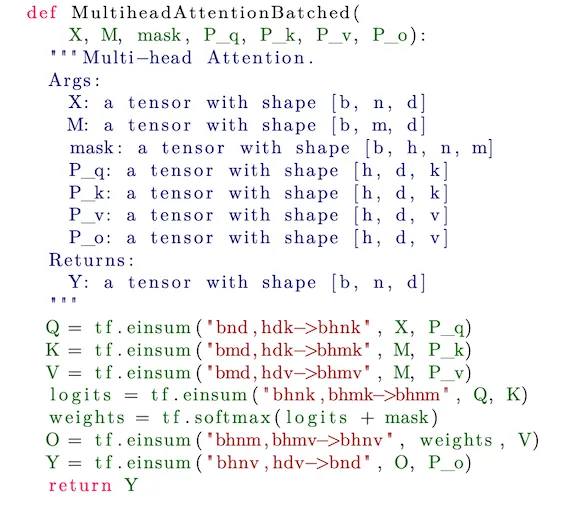### Transformer的复杂度和上下文长度

2个矩阵乘法$（a，b）\star（b，c）$的复杂度为$O（a \star b \star c）$

1. 线性投影得到$Q，K，V$：大小为$（n，d）$的嵌入矩阵乘以$h$个可学习矩阵$（d，k）$$（d，k）$$（d，v）$。因此，复杂度约为$O(nd^2)$

2. $Q$与变换后的$K$相乘，然后再乘以$V$$（n，k）\star（k，n）=（n，n）$，以及$（n，n）\star（n，v）=（n，v）$。复杂度约为$O(n^2d)$

$d>n$时（例如，在LLaMa中，$n=2K$$d=4K$），$O(nd^2)$这个术语非常重要。

$n>d$时（例如，在使用$n=65K$$d=4K$进行MosaicML训练时），$O(n^2d)$这个术语非常重要。

$2000^2=4000000, 100000^2=10000000000$

（1）$n$的复杂度为$M \star (n^2d + nd^2)$

（2）$p \star n$的复杂度为$\frac{M}{p} \star ((pn)^2d + (pn)d^2)$

## 增加上下文长度的优化技术

### [技巧1] 更好的位置编码——ALiBi

**这是因为位置正弦编码没有“外推（extrapolation）”能力。**在ALiBI论文中，作者表明，在推理过程中，位置正弦编码对于上下文窗口的扩展不具有健壮性，在增加了一些tokens后，性能开始下降。因此，缺乏“外推”能力基本上意味着在推理/微调过程中不能使用比训练时更大的上下文长度。关于“外推”的概念和各种位置编码的比较详见。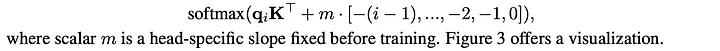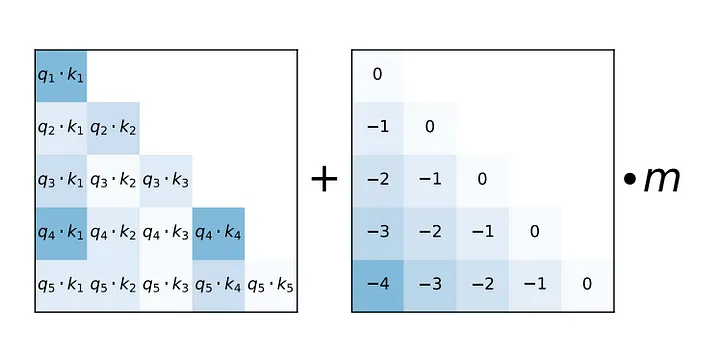### [技巧2] 稀疏注意力机制BigBird注意力分数方法结合了全局、局部和随机机制。在这篇论文中，作者展示了一个重要的观察结果，即在计算相似性分数和不同节点间的信息流动之间存在固有的张力（tension）关系（即一个token对其他tokens的影响能力）。

### [技巧3] FlashAttention——用于GPU的注意力层高效实现

1. $S = Q \star K$

2. $P = softmax(S)$

3. $O = P \star V$FlashAttention算法的主要思路是将输入的$Q$$K$$V$矩阵划分成块（block），将这些块从HBM加载至SRAM中，然后根据这些块来计算注意力输出。这个过程被称为“切片（tiling）”。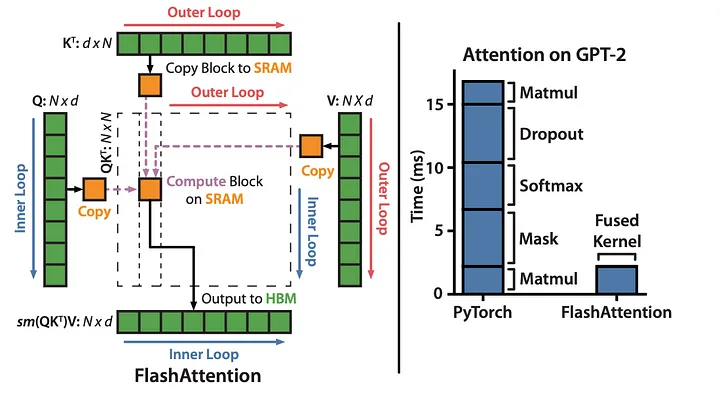“矩阵乘法”运算已经针对GPU进行了优化，可将FlashAttention算法视为针对GPU进行优化的“注意力层”运算的实现。作者通过切片和优化HBM访问，融合了多个乘法和softmax操作。

### [技巧5] 条件计算

$d > n$时，速度瓶颈不在注意力层，而是在前馈层（feedforward）和投影层(参考苏神博客)。减少浮点运算的常见方法是采用某种条件计算，避免将所有模型参数应用于输入序列的所有tokens。

“轻型和重型前馈分支仅在其隐藏层维度上有所不同，其中轻型分支的隐藏层维度小于标准T5前馈层，而重型分支的隐藏维度更高。”